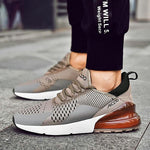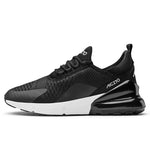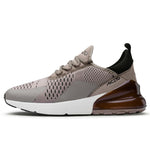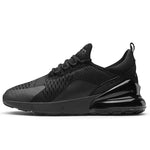Sale
••••# Alexis Shoes

- Breathable, two-toned casual sneaker

- Upper Material: Mesh (Air mesh)
- Closure Type: Lace-Up
- Outsole Material: Rubber
- Lining Material: Cotton Fabric
- Insole Material: EVA

-Feature: Breathable, Height Increasing, Massage,
Sweat Absorbant, Light, Hard-Wearing, Anti-Odor

US Sizes:     Foot Length
7        =  39      = 24.5cm
7.5     =  40      = 25.0cm
8        =  41      = 25.5cm
8.5     =  42      = 26.5cm
9.5     =  43      = 27.0cm
10      =  44      = 27.5cm
11      =  45      = 28.5cm
12      =  46      = 29.0cm